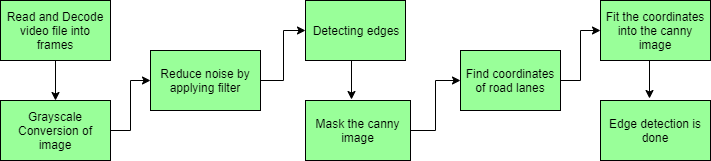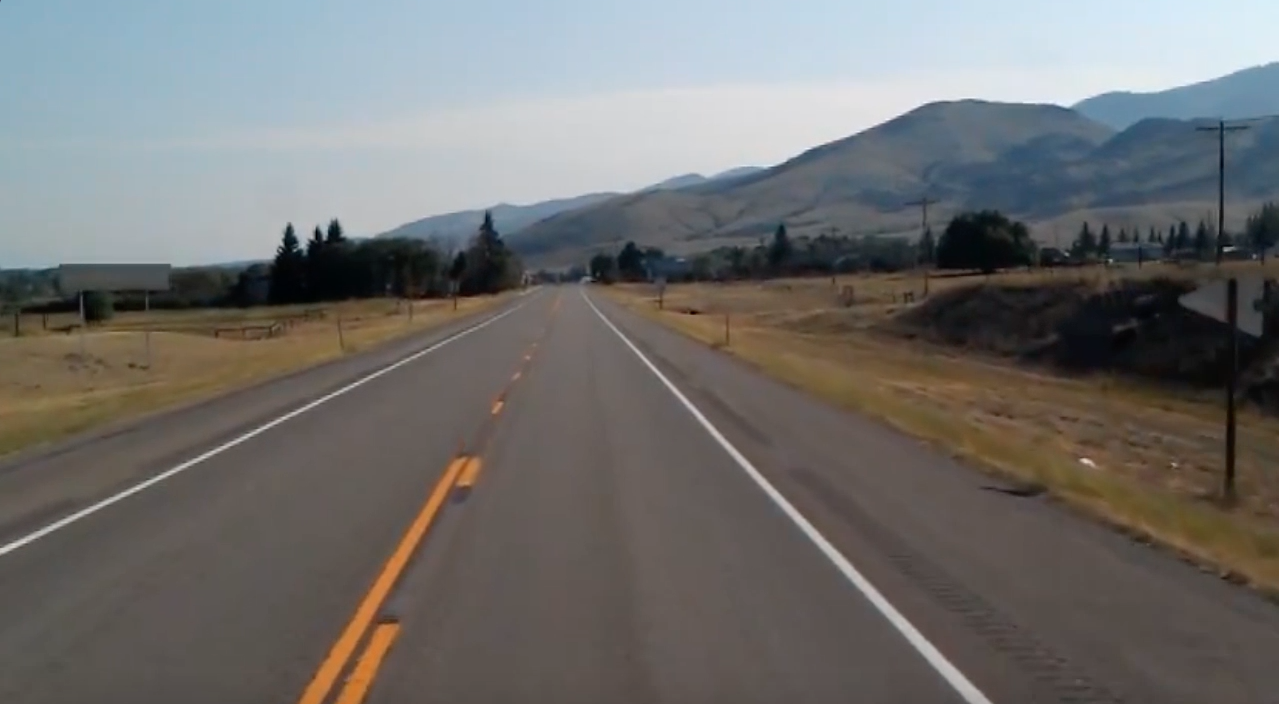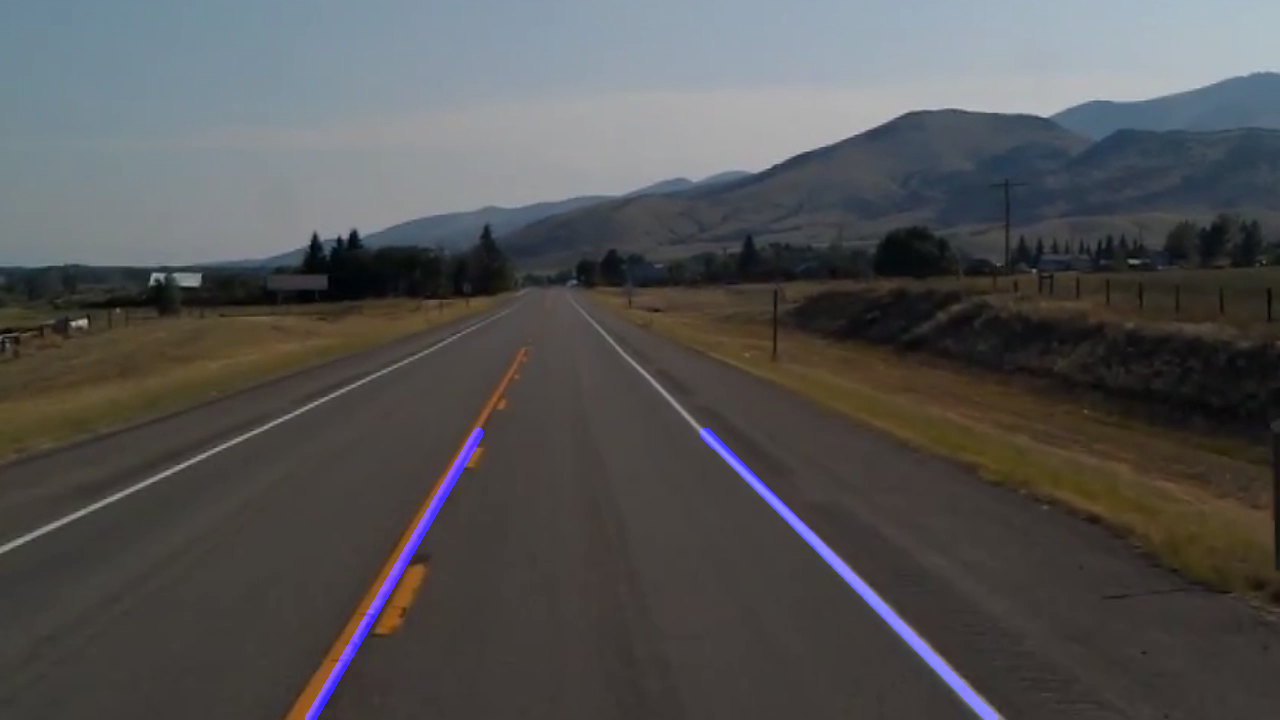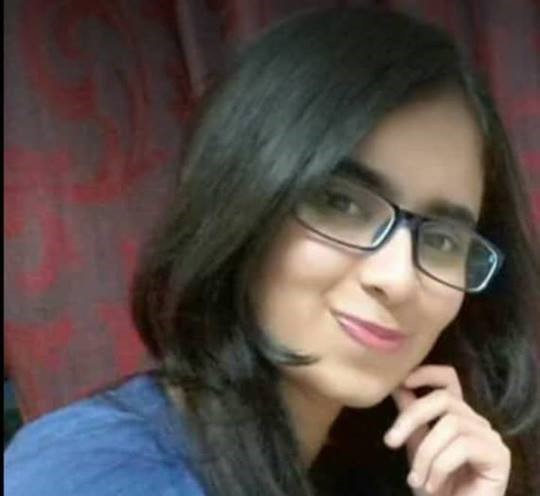# OpenCV | Real Time Road Lane Detection

Introduction

Autonomous Driving Car is one of the most disruptive innovations in AI. Fuelled by Deep Learning algorithms, they are continuously driving our society forward and creating new opportunities in the mobility sector. An autonomous car can go anywhere a traditional car can go and does everything that an experienced human driver does. But it’s very essential to train it properly. One of the many steps involved during the training of an autonomous driving car is lane detection, which is the preliminary step. Today, we are going to learn how to perform lane detection using videos.

Lane detection involves the following steps:• Capturing and decoding video file: We will capture the video using VideoCapture object and after the capturing has been initialized every video frame is decoded (i.e. converting into a sequence of images).
• Grayscale conversion of image: The video frames are in RGB format, RGB is converted to grayscale because processing a single channel image is faster than processing a three-channel colored image.
• Reduce noise: Noise can create false edges, therefore before going further, it’s imperative to perform image smoothening. Gaussian filter is used to perform this process.
• Canny Edge Detector: It computes gradient in all directions of our blurred image and traces the edges with large changes in intesity. For more explanation please go through this article: Canny Edge Detector
• Region of Interest: This step is to take into account only the region covered by the road lane. A mask is created here, which is of the same dimension as our road image. Furthermore, bitwise AND operation is performed between each pixel of our canny image and this mask. It ultimately masks the canny image and shows the region of interest traced by the polygonal contour of the mask.
• Hough Line Transform: The Hough Line Transform is a transform used to detect straight lines. The Probabilistic Hough Line Transform is used here, which gives output as the extremes of the detected lines

Dataset: The dataset consists of the video file of a road.

Now let’s start the implementation process:

• NumPy: It comes by default with anaconda
• Matplotlib: To install matplotlib, type – “pip install matplotlib” into your command line
• OpenCV: It can be installed in two ways, using anaconda or using pip.
To install using anaconda, type- “conda install -c conda-forge opencv”, or to install using pip, type-
“pip install opencv-python” into your command line
•  `# Import the required libraries ` `import` `cv2 ` `import` `numpy as np ` `import` `matplotlib.pyplot as plt `

The canny function calculates derivative in both x and y directions, and according to that, we can see the changes in intensity value. Larger derivatives equal to High intensity(sharp changes), Smaller derivatives equal to Low intensity(shallow changes):

 `def` `canny_edge_detector(image): ` `     `  `    ``# Convert the image color to grayscale ` `    ``gray_image ``=` `cv2.cvtColor(image, cv2.COLOR_RGB2GRAY)  ` `     `  `    ``# Reduce noise from the image ` `    ``blur ``=` `cv2.GaussianBlur(gray_image, (``5``, ``5``), ``0``)  ` `    ``canny ``=` `cv2.Canny(blur, ``50``, ``150``) ` `    ``return` `canny `

Masking our canny image after finding the region of interest:

 `def` `region_of_interest(image): ` `    ``height ``=` `image.shape[``0``] ` `    ``polygons ``=` `np.array([ ` `        ``[(``200``, height), (``1100``, height), (``550``, ``250``)] ` `        ``]) ` `    ``mask ``=` `np.zeros_like(image) ` `     `  `    ``# Fill poly-function deals with multiple polygon ` `    ``cv2.fillPoly(mask, polygons, ``255``)  ` `     `  `    ``# Bitwise operation between canny image and mask image ` `    ``masked_image ``=` `cv2.bitwise_and(image, mask)  ` `    ``return` `masked_image `

We are going to find the coordinates of our road lane:

 `def` `create_coordinates(image, line_parameters): ` `    ``slope, intercept ``=` `line_parameters ` `    ``y1 ``=` `image.shape[``0``] ` `    ``y2 ``=` `int``(y1 ``*` `(``3` `/` `5``)) ` `    ``x1 ``=` `int``((y1 ``-` `intercept) ``/` `slope) ` `    ``x2 ``=` `int``((y2 ``-` `intercept) ``/` `slope) ` `    ``return` `np.array([x1, y1, x2, y2]) `

Differentiating left and right road lanes with the help of positive and negative slopes respectively and appending them into the lists, if the slope is negative then the road lane belongs to the left-hand side of the vehicle, and if the slope is positive then the road lane belongs to the right-hand side of the vehicle:

 `def` `average_slope_intercept(image, lines): ` `    ``left_fit ``=` `[] ` `    ``right_fit ``=` `[] ` `    ``for` `line ``in` `lines: ` `        ``x1, y1, x2, y2 ``=` `line.reshape(``4``) ` `         `  `        ``# It will fit the polynomial and the intercept and slope ` `        ``parameters ``=` `np.polyfit((x1, x2), (y1, y2), ``1``)  ` `        ``slope ``=` `parameters[``0``] ` `        ``intercept ``=` `parameters[``1``] ` `        ``if` `slope < ``0``: ` `            ``left_fit.append((slope, intercept)) ` `        ``else``: ` `            ``right_fit.append((slope, intercept)) ` `             `  `    ``left_fit_average ``=` `np.average(left_fit, axis ``=` `0``) ` `    ``right_fit_average ``=` `np.average(right_fit, axis ``=` `0``) ` `    ``left_line ``=` `create_coordinates(image, left_fit_average) ` `    ``right_line ``=` `create_coordinates(image, right_fit_average) ` `    ``return` `np.array([left_line, right_line]) `

Fitting the coordinates into our actual image and then returning the image with the detected line(road with the detected lane):

 `def` `display_lines(image, lines): ` `    ``line_image ``=` `np.zeros_like(image) ` `    ``if` `lines ``is` `not` `None``: ` `        ``for` `x1, y1, x2, y2 ``in` `lines: ` `            ``cv2.line(line_image, (x1, y1), (x2, y2), (``255``, ``0``, ``0``), ``10``) ` `    ``return` `line_image `

Firstly, the video file is read and decoded into frames and using Houghline method the straight line which is going through the image is detected. Then we call all the functions.

 `# Path of dataset directory ` `cap ``=` `cv2.VideoCapture(``"datasets\test2.mp4"``)  ` `while``(cap.isOpened()): ` `    ``_, frame ``=` `cap.read() ` `    ``canny_image ``=` `canny_edge_detector(frame) ` `    ``cropped_image ``=` `region_of_interest(canny_image) ` `     `  `    ``lines ``=` `cv2.HoughLinesP(cropped_image, ``2``, np.pi ``/` `180``, ``100``,  ` `                            ``np.array([]), minLineLength ``=` `40``,  ` `                            ``maxLineGap ``=` `5``)  ` `     `  `    ``averaged_lines ``=` `average_slope_intercept(frame, lines)  ` `    ``line_image ``=` `display_lines(frame, averaged_lines) ` `    ``combo_image ``=` `cv2.addWeighted(frame, ``0.8``, line_image, ``1``, ``1``)  ` `    ``cv2.imshow(``"results"``, combo_image) ` `     `  `    ``# When the below two will be true and will press the 'q' on ` `    ``# our keyboard, we will break out from the loop ` `     `  `    ``# # wait 0 will wait for infinitely between each frames.  ` `    ``# 1ms will wait for the specified time only between each frames ` `    ``if` `cv2.waitKey(``1``) & ``0xFF` `=``=` `ord``(``'q'``):       ` `        ``break` ` `  `# close the video file ` `cap.release()  ` ` `  `# destroy all the windows that is currently on ` `cv2.destroyAllWindows()  `

Input :Output :